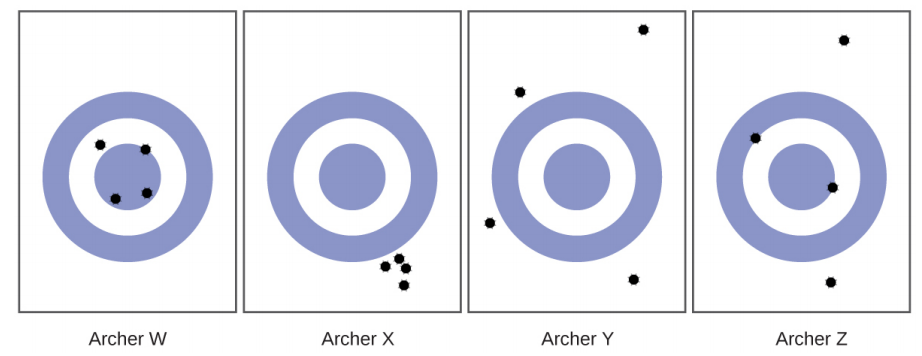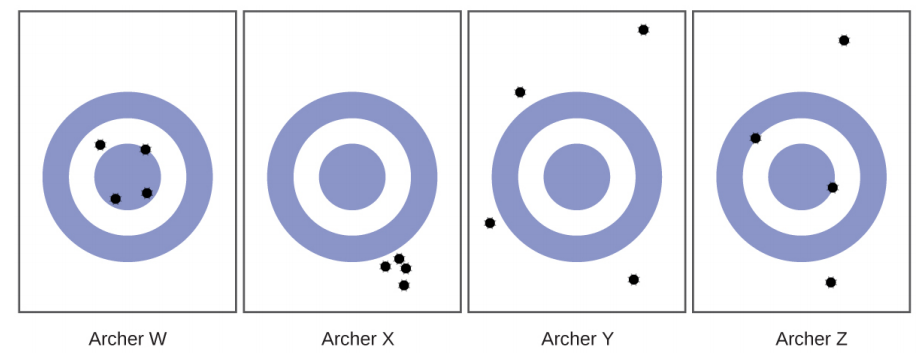# Problem: Consider the results of the archery contest shown in this figure.(b) Which archer is most accurate?

###### FREE Expert Solution

We’re being asked to determine which archer is most accurateRecall that:

• Accuracy refers to how close the measurement is to the ‘true/actual value’.

• Precision refers to how close the measurements are to each other.

For this problem, the true value is the center violet circle

Let’s analyze each archer:

98% (263 ratings)###### Problem Details

Consider the results of the archery contest shown in this figure.

(b) Which archer is most accurate?Frequently Asked Questions

What scientific concept do you need to know in order to solve this problem?

Our tutors have indicated that to solve this problem you will need to apply the Accuracy & Precision concept. If you need more Accuracy & Precision practice, you can also practice Accuracy & Precision practice problems.

What professor is this problem relevant for?

Based on our data, we think this problem is relevant for Professor Maxwell's class at UCF.

What textbook is this problem found in?

Our data indicates that this problem or a close variation was asked in Chemistry - OpenStax 2015th Edition. You can also practice Chemistry - OpenStax 2015th Edition practice problems.